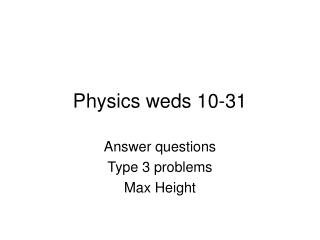DownloadDownload PresentationPhysics weds 10-31

# Physics weds 10-31

Télécharger la présentation## Physics weds 10-31

- - - - - - - - - - - - - - - - - - - - - - - - - - - E N D - - - - - - - - - - - - - - - - - - - - - - - - - - -
##### Presentation Transcript

1. Physics weds 10-31 Answer questions Type 3 problems Max Height

2. Challenge problem • A rude tourist throws a peach pit horizontally with 7.0 m/s velocity out of an elevator cage. The elevator is moving upward with a constant velocity of 8.5 m/s velocity. • How long will it take the peach pit to land on the ground, 17.0 m below his initial position?

3. Type 3 problems • Initial velocity at angle • Final position is not equal to start position (vertically) • Solve for time using quadratic formula or Solve for vertical final velocity first, then time

4. Type 3 problems • Firefighters are using water from hose to put out a fire 13.5 m above the ground. The nozzle of the hose stands 0.75 m above the ground. • If the water comes out 44.5 m/s at 49° above the horizontal, how far away from the building should the firefighters stand?

5. Steps to solutions • Determine the givens and fill them into the chart • Use trigonometry to find the initial velocities • Usually solve for the final vertical velocity’ • Then solve for time • Then solve for anything else needed

6. Max height • Max height is the maximum vertical displacement for a projectile • Calculate the from the vertical position of launch to the point where the object no longer moves upward.

7. Maximum height • Occurs at the apex of the arc. • The vertical velocity at that point is _____ • Calculate using the vertical information at the max height • Put a third column in chart • Title “max v” • The “d” in the max v column is the max height from surface • The time is NOT the same as time in the “H” or “V” column

8. If object is shot off the cliff… • Add the height of cliff to max height to get the height of object above the ground

9. Max height question set up • Angle _______ • Initial velocity ____ • Ledge of Cliff? _____ • Height ______

10. A Halloween monster question • Create a question with a Halloween theme that centers on projectile motion. • Any type of projectile motion is allowable • The question must ask for 2 different unknowns (such as time and the Maximum height) • The numbers do not have to be realistic